Courses

# Test: Seating Arrangement- 4

## 15 Questions MCQ Test Integrated Reasoning for GMAT | Test: Seating Arrangement- 4

Description
This mock test of Test: Seating Arrangement- 4 for LR helps you for every LR entrance exam. This contains 15 Multiple Choice Questions for LR Test: Seating Arrangement- 4 (mcq) to study with solutions a complete question bank. The solved questions answers in this Test: Seating Arrangement- 4 quiz give you a good mix of easy questions and tough questions. LR students definitely take this Test: Seating Arrangement- 4 exercise for a better result in the exam. You can find other Test: Seating Arrangement- 4 extra questions, long questions & short questions for LR on EduRev as well by searching above.
QUESTION: 1

### Directions to Solve A, B, C, D, E, F and G are sitting in a row facing North : F is to the immediate right of E. E is 4th to the right of G. C is the neighbour of B and D. Person who is third to the left of D is at one of ends. Question - Who are to the left of C ?

Solution: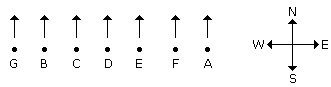G an B are to the left of C.

QUESTION: 2

### Directions to Solve A, B, C, D, E, F and G are sitting in a row facing North : F is to the immediate right of E. E is 4th to the right of G. C is the neighbour of B and D. Person who is third to the left of D is at one of ends. Question - Which of the following statement is not true ?

Solution:QUESTION: 3

### Directions to Solve A, B, C, D, E, F and G are sitting in a row facing North : F is to the immediate right of E. E is 4th to the right of G. C is the neighbour of B and D. Person who is third to the left of D is at one of ends. Question - Who are the neighbours of B ?

Solution:C and G are the neighbours of B.

QUESTION: 4
Directions to Solve
A, B, C, D, E, F and G are sitting in a row facing North :
1. F is to the immediate right of E.
2. E is 4th to the right of G.
3. C is the neighbour of B and D.
4. Person who is third to the left of D is at one of ends.
Question -

What is the position of A ?

Solution:A is in extreme right.

QUESTION: 5
Directions to Solve
Each of these questions are based on the information given below :
1. 8 persons E, F, G, H, I, J, K and L are seated around a square table - two on each side.
2. There are 3 ladies who are not seated next to each other.
3. J is between L and F.
4. G is between I and F.
5. H, a lady member is second to the left of J.
6. F, a male member is seated opposite to E, a lady member.
7. There is a lady member between F and I.
Question -

Who among the following is to the immediate left of F ?

Solution: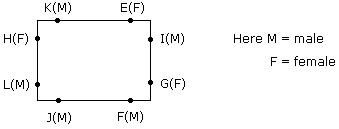J is to the immediate left of F.

QUESTION: 6
Directions to Solve
Each of these questions are based on the information given below :
1. 8 persons E, F, G, H, I, J, K and L are seated around a square table - two on each side.
2. There are 3 ladies who are not seated next to each other.
3. J is between L and F.
4. G is between I and F.
5. H, a lady member is second to the left of J.
6. F, a male member is seated opposite to E, a lady member.
7. There is a lady member between F and I.
Question -

What is true about J and K ?

Solution:Both are male.

QUESTION: 7
Directions to Solve
Each of these questions are based on the information given below :
1. 8 persons E, F, G, H, I, J, K and L are seated around a square table - two on each side.
2. There are 3 ladies who are not seated next to each other.
3. J is between L and F.
4. G is between I and F.
5. H, a lady member is second to the left of J.
6. F, a male member is seated opposite to E, a lady member.
7. There is a lady member between F and I.
Question -

How many persons are seated between K and F ?

Solution:Three persons are seated between K and F(H, L and J) or E, I and G.

QUESTION: 8
Directions to Solve
Each of these questions are based on the information given below :
1. 8 persons E, F, G, H, I, J, K and L are seated around a square table - two on each side.
2. There are 3 ladies who are not seated next to each other.
3. J is between L and F.
4. G is between I and F.
5. H, a lady member is second to the left of J.
6. F, a male member is seated opposite to E, a lady member.
7. There is a lady member between F and I.
Question -

Who among the following are three lady members ?

Solution:The three lady members are E, H and G.

QUESTION: 9
Directions to Solve
Each of these questions are based on the information given below :
1. 8 persons E, F, G, H, I, J, K and L are seated around a square table - two on each side.
2. There are 3 ladies who are not seated next to each other.
3. J is between L and F.
4. G is between I and F.
5. H, a lady member is second to the left of J.
6. F, a male member is seated opposite to E, a lady member.
7. There is a lady member between F and I.
Question -

Who among the following is seated between E and H ?

Solution:K is seated between E and H.

QUESTION: 10

Directions to Solve

In a class there are seven students (including boys and girls) A, B, C, D, E, F and G. They sit on three benches I, II and III. Such that at least two students on each bench and at least one girl on each bench. C who is a girl student, does not sit with A, E and D. F the boy student sits with only B. A sits on the bench I with his best friends. G sits on the bench III. E is the brother of C.

Question -

How many girls are there out of these 7 students ?

Solution: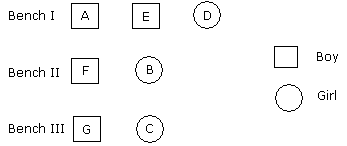The number of girls is either 3 or 4.

QUESTION: 11
Directions to Solve
In a class there are seven students (including boys and girls) A, B, C, D, E, F and G. They sit on three benches I, II and III. Such that at least two students on each bench and at least one girl on each bench. C who is a girl student, does not sit with A, E and D. F the boy student sits with only B. A sits on the bench I with his best friends. G sits on the bench III. E is the brother of C.
Question -

Who sits with C ?

Solution:G sits with C.

QUESTION: 12
Directions to Solve
In a class there are seven students (including boys and girls) A, B, C, D, E, F and G. They sit on three benches I, II and III. Such that at least two students on each bench and at least one girl on each bench. C who is a girl student, does not sit with A, E and D. F the boy student sits with only B. A sits on the bench I with his best friends. G sits on the bench III. E is the brother of C.
Question -

On which bench there are three students ?

Solution:There are three students in Bench I.

QUESTION: 13

Directions to Solve

In a class there are seven students (including boys and girls) A, B, C, D, E, F and G. They sit on three benches I, II and III. Such that at least two students on each bench and at least one girl on each bench. C who is a girl student, does not sit with A, E and D. F the boy student sits with only B. A sits on the bench I with his best friends. G sits on the bench III. E is the brother of C.

Question -

Which of the following is the group of girls ?

Solution:BCD are the group of girls.

QUESTION: 14

Directions to Solve

Six girls are sitting in a circle facing to the centre of the circle. They are P, Q, R, S, T and V. T is not between Q and S but some other one. P is next to the left of V. R is 4th to the right of P.

Question -

Which of the following statement is not true ?

Solution: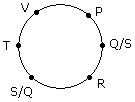QUESTION: 15
Directions to Solve
Six girls are sitting in a circle facing to the centre of the circle. They are P, Q, R, S, T and V. T is not between Q and S but some other one. P is next to the left of V. R is 4th to the right of P.
Question -

If P and R interchange their positions then which of the following pair will sit together ?

Solution:

After changing the position of P and R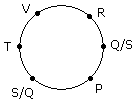V and T are together.